# Explaining Dark Matter and Dark Energy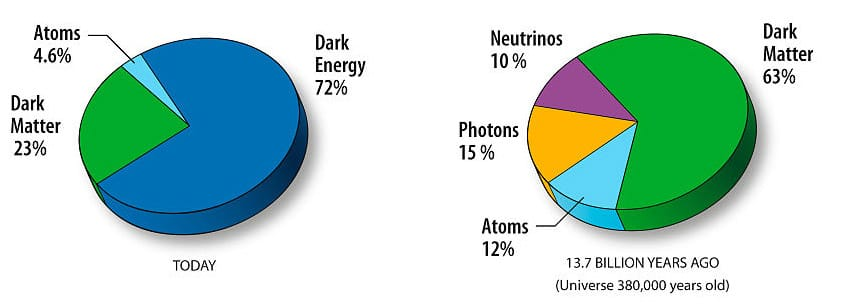In the present-day, about 14 billion years since the Big Bang, the energy composition of the Universe is approximately the following:

A small amount of atoms and light
A medium amount of dark matter
A large amount of dark energy

However, after right after its initial inflationary period, the makeup of the Universe was roughly:

A medium amount of atoms and light
A large amount of dark matter
No dark energy

Also note that there also seems to be a generally constant 6:1 proportion of dark matter to atoms (matter with mass).

I’m going to provide a model that explains these general observations. The model is created by referencing the relationship patterns described in Theory of Thought.

Prior to the Big Bang, there was a perfectly ordered singularity of space and time that contained all the energy we have in our present-day Universe.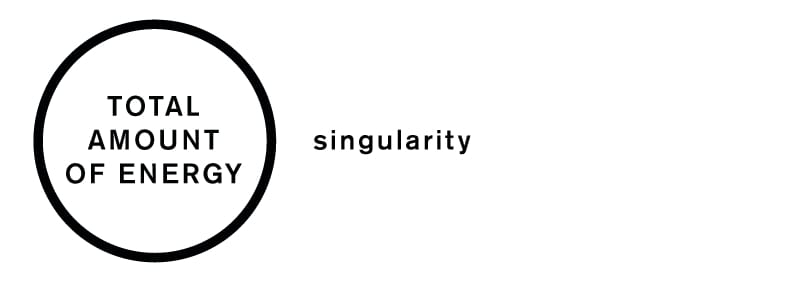Since the singularity, the amount of energy within the Universe has remained constant.

And we know the Universe has been expanding ever since the Big Bang, and we also know that the rate of expansion is increasing (ie. exponential rate).

Instead of ‘inflating’ the circle, I’m going to illustrate expansion by adding circles within circles, that create layers, which in turn creates ‘depth’.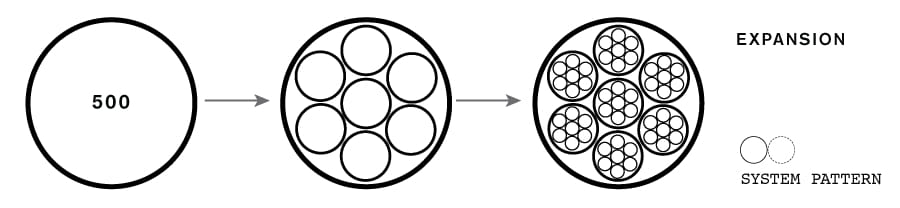So where are these circles coming from? Time… Time creates these circles in response to ‘events in time’. So in effect, adding circles signifies increasing entropy, and the forward flow of time.

So how does this apply to dark Matter and dark Energy?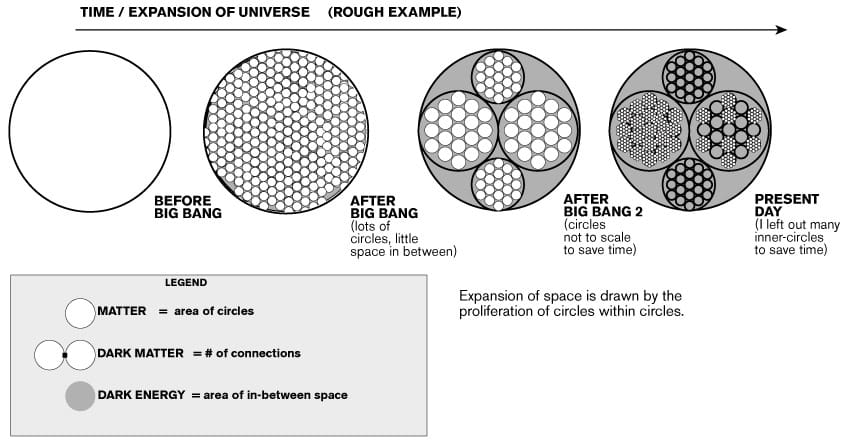In my very rudimentary model, the total amount of dark energy is proportional to the total amount of layers, while the amount of dark matter is proportional to the total number of ‘kissing’ circles.

By the way, the kissing number is 6: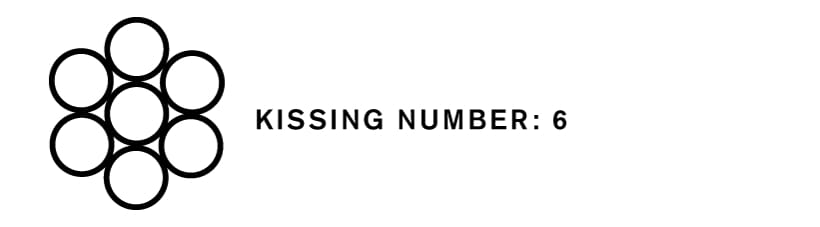The kissing number might be the maximum possible ratio between observed quantities of dark matter to matter, if it could be shown that these mysterious phenomenons have everything to do with circle packing.

And since each circle represents regular matter, dark matter is a function of the interconnectedness of matter, which gains in strength when there is lots of it grouped together... Hence why dark matter is important when considering galactic bodies rather than when considering small objects like atoms.

Now lets take a look at these ideas using the hierarchical pattern.

Watch this video to see the difference between the system and hierarchical pattern:

Within a hierarchical pattern, the flow of time adds new circles to the hierarchy.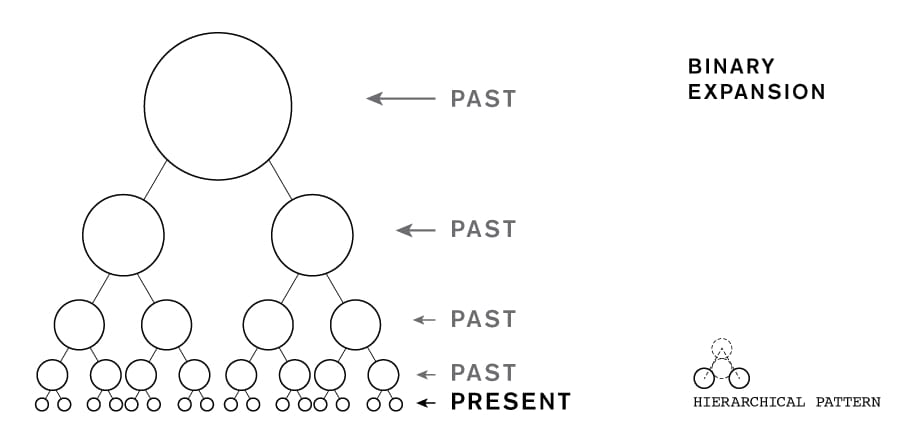And this is how dark energy and matter would be interpreted in the hierarchical pattern: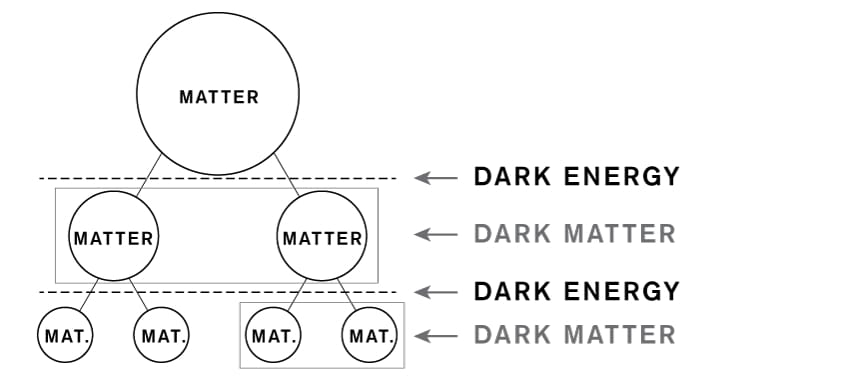And since the present is the bottom most layer of circles, the upper layers have essentially gone ‘dark’ - yet they are still important when understanding the configuration of total energy.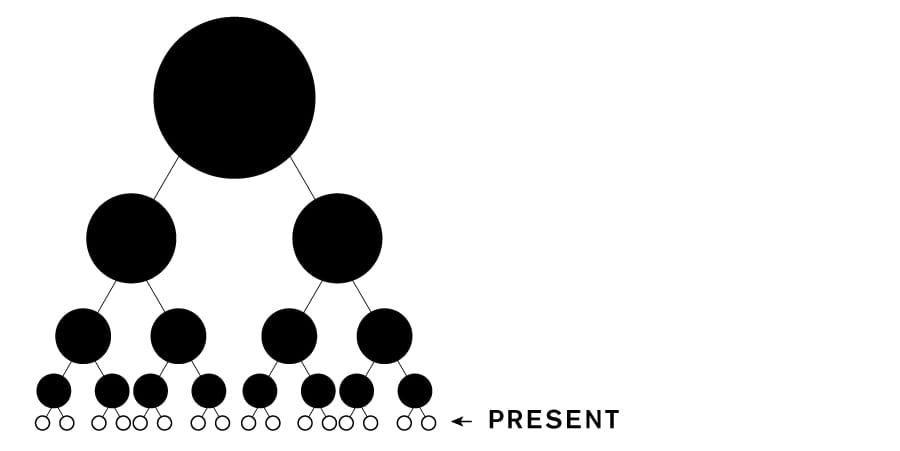Over time, the total area of the most inner-layer of circles, compared to the area of the ‘dark’ circles, shrinks, signifying a drop in the total matter to dark energy ratio.

The hierarchical expansion is also growing exponentially, which might explain how the Universe's speed of expansion is increasing.

Over time, the expansion of the universe creates lots of circles within circles - which unlike this diagram, are probably not so homogenous. So 13-14 billion years later, the universe finds itself somewhere within this circles within circles illustration, and we end up with the matter/energy makeup that is currently observed.

And there you have it! A simple, hypothetical model explaining the conceptual origin of matter, dark matter, and dark energy.

Note: I’m using a VERY SIMPLISTIC model. It is not drawn exactly, and is only meant to conceptualize 3 types of energy. Perhaps an illustration showing their exact progression over time might be done using my approach, but it would require a much more precise measurement of areas, numbers of circles, packing configuration of circles, and so forth.

Now learn how the hierarchical pattern, entropy and Pascal’s Triangle fit together!